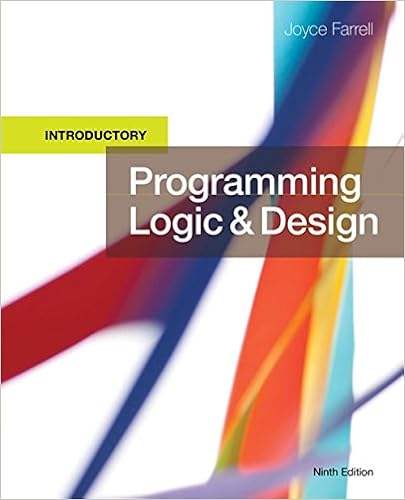# Dice2 randomlimit output prompt input guess return

• Homework Help
• 53
• 95% (221) 210 out of 221 people found this document helpful

This preview shows page 50 - 53 out of 53 pages.

##### We have textbook solutions for you!
The document you are viewing contains questions related to this textbook.The document you are viewing contains questions related to this textbook.
Chapter 5 / Exercise 12
Programming Logic and Design, Introductory
FarrellExpert Verified
3.Create the logic for the dice game Pig, in which a player can compete with the computer. The object of the game is to be the first to score 100 points. The user and
##### We have textbook solutions for you!
The document you are viewing contains questions related to this textbook.The document you are viewing contains questions related to this textbook.
Chapter 5 / Exercise 12
Programming Logic and Design, Introductory
FarrellExpert Verified
Programming Logic and Design, 8eSolutions 5-51When the computer does not roll a 1 and can choose whether to roll again, generate a random value of 1 or 2. The computer will then decide to continue when the value is 1 and decide to quit and pass the turn to the player when the value is not 1.Answer: A sample solution is as follows:Pseudocode:startDeclarationsnum dice1num dice2num userCountnum computerCountnum userTurnnum LIMIT = 2num WIN_NUM =100housekeeping()while computerCount < WIN_NUM AND userCount < WIN_NUMdetailLoop()endwhilestophousekeeping()output ”The first to 100 wins the game of Pig”userCount = 0computerCount = 0userTurn = 1returndetailLoop()if userTurn = 1 thenoutput “User’s turn to roll the dice”elseoutput “Computer’s turn to roll the dice”endifdice1 = random(LIMIT)dice2 = random(LIMIT)if userTurn = 1 thenif dice1 = 1 OR dice2 = 1 thenoutput “Computer's turn”userTurn = 0if dice1 = 1 AND dice2 = 1 thenuserCount = 0endifelseuserCount = userCount + dice1 + dice2if userCount >= WIN_NUMoutput “User wins!”elseoutput “Enter a 1 to play again or 0 to pass”
Programming Logic and Design, 8eSolutions 5-52input userTurnendifendifelse if dice1 = 1 OR dice2 = 1output “User's turn”userTurn = 1if dice1 = 1 AND dice2 = 1 THENcomputerCount = 0endifelsecomputerCount = computerCount + dice1 + dice2if computerCount >= WIN_NUMoutput “Computer wins!”elseif random(LIMIT) = 1 thenuserTurn = 0elseuserTurn = 1endifendifendifendifreturnfinishUp()output “End of program”returnFlowchart:The flowchart’s structure will be very similar to that given for previous exercises.Up for Discussion1.Suppose you wrote a program that you suspect is in an infinite loop because it keeps running for several minutes with no output and without ending. What would you add to your program to help you discover the origin of the problem?
•••